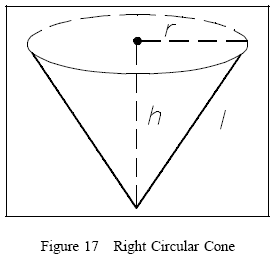# the radius and height of a cone are in the ratio 4:3.tha area of the base is 154cm2.find the area of the curved surface area.

It is a easy question.

We have given the ratio of radius and height i.e 4 : 3

Let

Height = 3x

Slant Height = root [(4x)2 +(3x)2 ]=5x {Pythagoreus Theorem}We know that

Area of Base = pie X radius

154=22/7 X 16x2

--->x=1.75cm